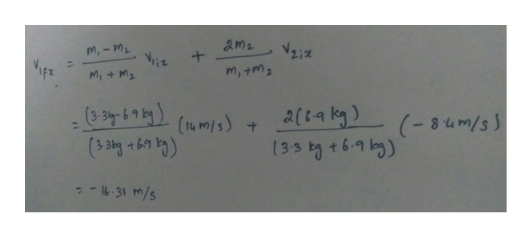# Two balls moving in opposite directions have velocities v1ix= 14ˍm/s and v2ix = -8.4ˍm/s . If their masses are m1 = 3.3ˍkg and m2 = 6.9ˍkg, respectively, and if their collision is perfectly elastic, then predict the velocity of each ball following the collision.

Question
40 views

Two balls moving in opposite directions have velocities v1ix= 14ˍm/s and v2ix = -8.4ˍm/s . If their masses are m1 = 3.3ˍkg and m2 = 6.9ˍkg, respectively, and if their collision is perfectly elastic, then predict the velocity of each ball following the collision.

check_circle

Step 1

Write an expression velocity of the fir...help_outlineImage Transcriptioncloseams m,-mi M,+M m, +ms (3-3y-6-9 2(64 kg) 135 병 +6-9 5) (-84m/s 16-31 m/s fullscreen

### Want to see the full answer?

See Solution

#### Want to see this answer and more?

Solutions are written by subject experts who are available 24/7. Questions are typically answered within 1 hour.*

See Solution
*Response times may vary by subject and question.
Tagged in

### Physics